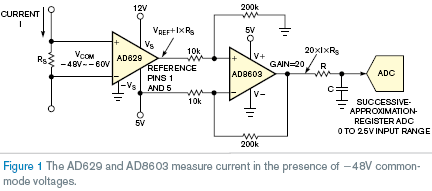# Single-Supply Circuit Measures -48 V High-Side Current

The nominal –48V rail, which finds wide use in wireless base stations and other telecommunications equipment in network central offices, can vary from –48 to –60V. Measuring its current draw typically requires components that operate on ±15V dual supplies. Eliminating the negative supply would reduce system complexity and cost. This Design Idea uses an AD629 difference amplifier and an AD8603 operational amplifier, both from Analog Devices, to measure current at –48 to –60 V and operates from a single positive-power supply.Figure 1 shows how the AD629 and AD8603 measure current in the presence of a –48 V common-mode voltage. The following equations demonstrate how the AD629 difference amplifier can condition voltages beyond its supply ranges: VCOM_MAX=20×(VS–1.2)–19×VREF, and VCOM_MIN=20×(–VS+1.2)–19×VREF. With a 5V reference, the common-mode input range is –71 to +121V. The current, I, flows through the shunt resistor, RS, causing a differential voltage, which the difference amplifier senses. The AD629 has a fixed gain of one, so the output voltage is I×RS+VREF. The AD8603 functions as a subtractor so that it can reject the common-mode voltage, VREF, and apply gain to the signal of interest, I×RS. A factor of 20 amplifies the signal to span the 2.5V full-scale range of the ADC.

This Design Idea uses the AD8603 because it has low input-bias current and low offset drift. In addition, the rail-to-rail output allows it to share the same supply as the ADC. In this stage, the subtractor rejects the 5V common-mode signal from the voltage reference. The four resistors that form the subtractor must have matched ratios to obtain maximum common-mode rejection. If you cannot obtain tightly matched resistors, you can use an AD623 single-supply instrumentation amplifier in place of the AD8603, ensuring high common-mode rejection.

Offset, input-bias-current, and common-mode-rejection errors from both amplifiers result in a 163-mV maximum error at the output of the AD8603. This calculation assumes resistors with a 0.01% ratio match. The circuit was verified on the bench using 50-, 100-, and 200-mΩ shunts for RS.

EDN